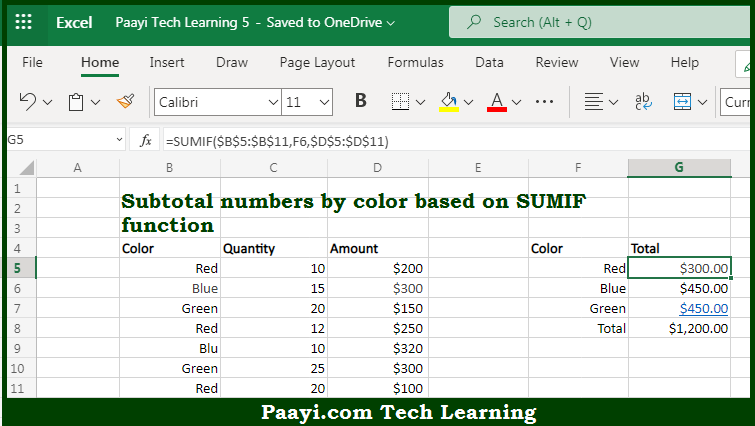# Learn How to SUBTOTAL By Color in Microsoft Excel

Written by | 0 Comments | 603 Views

In this article, you will learn how to COUNT various things in Microsoft Excel using a single/combination(s) of functions. You will also know how to SUBTOTAL By Color and see the generic formula.

SUBTOTAL By Color in Microsoft Excel

The main purpose of this formula is to subtotal numbers by color. Here we will learn how to subtotal numbers by color easily in Microsoft Excel. That implies, with the help of a formula based on the SUMIF function you can able to subtotal numbers by color. So, with the help of this formula, you can able to subtotal numbers by color easily in Microsoft Excel.

General Formula to SUBTOTAL By Color

=SUMIF(color_range,criteria,number_range)

The Explanation for the SUBTOTAL By ColorSo we know that with the help of the given formula above you can able to subtotal numbers by color. Here we will learn how to subtotal numbers by color easily in Microsoft Excel. As we know that the SUMIF function takes three arguments which are range, criteria, and sum_range. In the example provided here, we are using the range. So, with the help of this formula, you can able to able to subtotal numbers by color easily in Microsoft Excel.